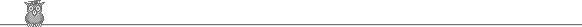18th Putnam 1958Problem A3

A sequence of numbers αi ∈ [0, 1] is chosen at random. Show that the expected value of n, where ∑1n αi > 1, ∑1n-1 αi ≤ 1 is e.

Solution

We can consider the possible values of α1, α2, ... , αn as points of the n-cube. The points corresponding to sum at most 1 are those in the corner at the origin, bounded by the hyperplane through (1, 0, ... , 0), (0, 1, 0, ... , 0) , ... , (0, ... , 0, 1). By an easy induction this has volume 1/(n-1)! . Let pn = the prob that the sum of the first n numbers is at most 1. We have just shown that pn = 1/(n-1)! .

Now the required expected value is (1/1! - 1/2!) 2 + (1/2! - 1/3!) 3 + (1/3! - 1/4!) 4 + ... = 2 + (3 - 2)/2! + (4 - 3)/3! + (5 - 4)/4! + ... = e.